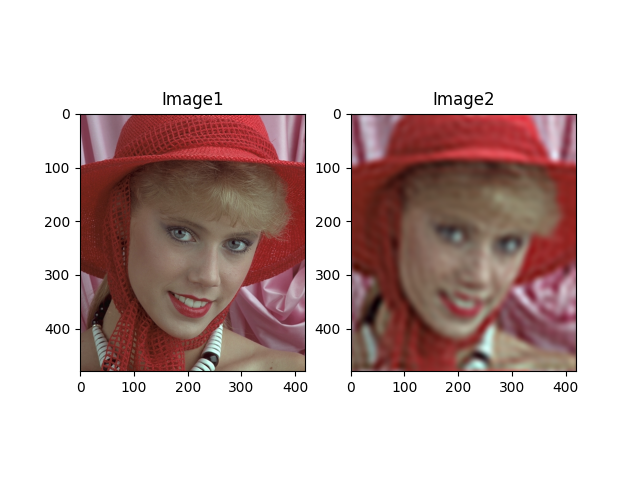# Image Filtering¶

Learn how to filter images through a pipeline.

```# Make sure we have the image plugin
import numpy as np
import imagepypelines as ip
ip.require('image')
```

Construct our filtering blocks

```# Frequency Filter
@ip.blockify()
def freq_filter(src, kernel):
return src * kernel

# Easy "frequency space" normalized lowpass filter
@ip.blockify()
x, y = np.meshgrid(np.linspace(-1, 1, shape), np.linspace(-1, 1, shape))
circle = np.sqrt(x*x + y*y)

if type=='low':
return np.where(circle > radius, 0, 1)

elif type=='high':
return np.where(circle > radius, 1, 0)

else:
raise ValueError("Filter 'type' must be either 'low' or 'high'")
```

Define our tasks and build the pipeline

```tasks = {
# set an entry point for images into the pipeline
'images': ip.Input(),
# get the image dimensions for later use
'shape': (ip.image.Dimensions(order="WHC"), 'images'),
# take the fourier transform
'fft': (ip.image.ImageFFT(order="WHC"), 'images'),
# produce 'frequency space' circular pass filters
'circles': (circular_pass_filter, 'shape'),
# add an extra dimension to the filters for broadcasting the arrays
'unsqueezed': (ip.image.Unsqueeze(-1), 'circles'),
# the convolution of your image and filter is element-wise
# multiplication in frequency space!!!
'filtered': (freq_filter, 'fft', 'unsqueezed'),
# take the inverse fourier transform of your filtered images
'ifft': (ip.image.ImageIFFT(order="WHC"), 'filtered'),
# Scale the images, cast dtype, and view the filtered images
# in sequence! Note: simple filtering in RGB results in artifacts!
'safe': (ip.image.DisplaySafe(), 'ifft'),
'null' : (ip.image.CompareView(pause_for=500), 'images','safe')
}

```

Out:

```images
shape
fft
circles
unsqueezed
filtered
ifft
safe
null
```

## Let’s process some data!¶

Let’s grab some example data from the ImagePypelines standard set

```images = [ip.image.panda(), ip.image.gecko(), ip.image.redhat()]
# Number and view the images!
processed = im_filt.process(images)
```Out:

```images
images
images
images
shape
fft
circles
unsqueezed
filtered
ifft
safe
null
```

Total running time of the script: ( 0 minutes 2.322 seconds)

Gallery generated by Sphinx-Gallery